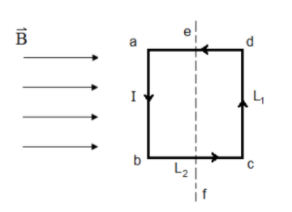# Problem: A rectangular loop with L1 = 0.15 m and L2 = 0.35 m is sitting in a magnetic field B = 0.35 T as shown in the figure. There is a counterclockwise current I = 0.55 A in the loop. Part (a) Express the torque of Fl ab, with respect of the axis ef, in terms of Fl and L2. Part (b) Express the torque of Fl on cd, with respect of the axis ef, in terms of Fl and L2.Part (c) What is the total torque on the current loop with respect of axis ef, in terms of F, and L2.

###### FREE Expert Solution

Part (a)

τab = F1L2/2

82% (458 ratings)###### Problem Details

A rectangular loop with L1 = 0.15 m and L2 = 0.35 m is sitting in a magnetic field B = 0.35 T as shown in the figure. There is a counterclockwise current I = 0.55 A in the loop.Part (a) Express the torque of Fl ab, with respect of the axis ef, in terms of Fl and L2

Part (b) Express the torque of Fl on cd, with respect of the axis ef, in terms of Fl and L2.

Part (c) What is the total torque on the current loop with respect of axis ef, in terms of F, and L2